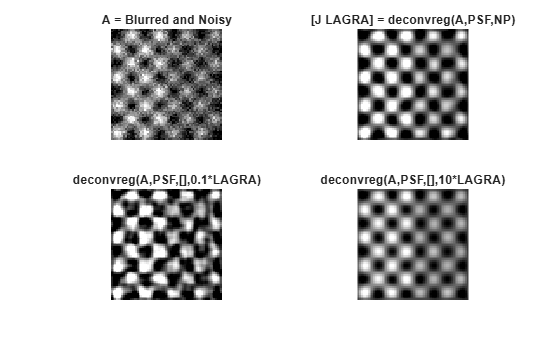# deconvreg

Deblur image using regularized filter

## Syntax

``J = deconvreg(I,psf)``
``J = deconvreg(I,psf,np)``
``J = deconvreg(I,psf,np,lrange)``
``J = deconvreg(I,psf,np,lrange,regop)``
``[J,lagra] = deconvreg(___)``

## Description

example

````J = deconvreg(I,psf)` deconvolves image `I` using the regularized filter algorithm, returning deblurred image `J`. The assumption is that the image `I` was created by convolving a true image with a point-spread function (PSF), `psf`, and possibly by adding noise. The algorithm is a constrained optimum in the sense of least square error between the estimated and the true images under requirement of preserving image smoothness.```
````J = deconvreg(I,psf,np)` specifies the additive noise power, `np`.```
````J = deconvreg(I,psf,np,lrange)` specifies the range, `lrange`, where the search for the optimal solution is performed. The algorithm finds an optimal Lagrange multiplier `lagra` within the `lrange` range.```
````J = deconvreg(I,psf,np,lrange,regop)` constrains the deconvolution using regularization operator `regop`. The default regularization operator is the Laplacian operator, to retain the image smoothness.```
````[J,lagra] = deconvreg(___)` outputs the value of the Lagrange multiplier, `lagra` in addition to the restored image, `J`.```

## Examples

collapse all

Create sample image.

`I = checkerboard(8);`

Create PSF and use it to create a blurred and noisy version of the input image.

```PSF = fspecial('gaussian',7,10); V = .01; BlurredNoisy = imnoise(imfilter(I,PSF),'gaussian',0,V); NOISEPOWER = V*prod(size(I));```

Deblur the image.

`[J LAGRA] = deconvreg(BlurredNoisy,PSF,NOISEPOWER);`

Display the various versions of the image.

```subplot(221); imshow(BlurredNoisy); title('A = Blurred and Noisy'); subplot(222); imshow(J); title('[J LAGRA] = deconvreg(A,PSF,NP)'); subplot(223); imshow(deconvreg(BlurredNoisy,PSF,[],LAGRA/10)); title('deconvreg(A,PSF,[],0.1*LAGRA)'); subplot(224); imshow(deconvreg(BlurredNoisy,PSF,[],LAGRA*10)); title('deconvreg(A,PSF,[],10*LAGRA)');```## Input Arguments

collapse all

Blurry image, specified as a numeric array of any dimension.

Data Types: `single` | `double` | `int16` | `uint8` | `uint16`

PSF, specified as a numeric array.

Data Types: `double`

Noise power, specified as a numeric scalar.

Data Types: `double`

Search range, specified as a numeric scalar or a 2-element numeric vector. If `lrange` is a scalar, then the algorithm assumes that `lagra` is equal to `lrange`. If you specify `lagra`, then the function ignores the `np` value

Data Types: `double`

Regularization operator, specified as a numeric array. The `regop` array dimensions must not exceed the dimensions of the image, `I`. Any nonsingleton dimensions must correspond to the nonsingleton dimensions of `psf`.

Data Types: `double`

## Output Arguments

collapse all

Deblurred image, returned as a numeric array. `J` has the same data type as `I`.

Lagrange multiplier, returned as a numeric scalar.

## Tips

• The output image `J` could exhibit ringing introduced by the discrete Fourier transform used in the algorithm. To reduce the ringing, use `I = edgetaper(I,psf)` before calling `deconvreg`.

 Gonzalez, R. C., and R. E. Woods. Digital Image Processing. Addison-Wesley Publishing Company, Inc., 1992.